cancel
Showing results for
Did you mean:

Fabric is Generally Available. Browse Fabric Presentations. Work towards your Fabric certification with the Cloud Skills Challenge.Helper III

## Looking for diff of two values by filters

kindly see the data set . there is cycle column with Q1,Q2,Q3. with ID , Amount column

I need difference of two cycle (which i'm selecting in filter) higher cycle will be base in difference

i attached dax, power bi view images also.

Dax which previously tried showing the difference(q3-q2-q1) which is working while im selecting q3 and q2 or q3 and q1.

but if im selecting q2 and q1 in filter . it should show q2 - q1 as answer. but this dax showing ( -q2-q1). here q2 should be base in difference.

highly appreciate for solutionplease see the data set with cycle column q3,q2,q1dax which actually giving q3-q2-q1 . but i need , if im selecting q2 and q1 in filter , it should show q2 - q1 . but it showing -q2-q1 .(higher cycle is base in difference)if im selecting q2 and q1 , q2 is higher order cycle ,so q2 is base in difference . so it should show q2-q1

2 ACCEPTED SOLUTIONSSuper User

Hello @sarath_chandra ,

Hope this measure helps:

``````result =
VAR current_id = SELECTEDVALUE ( 'Table'[ID] )
VAR current_cycle = SELECTEDVALUE ( 'Table 2'[Cycle] )
VAR t =
ALLSELECTED ( 'Table'[Cycle] ),
"@maxrank",
CALCULATE (
MAX ( 'Table 2'[Rank] ),
'Table 2'[Cycle] IN ALLSELECTED ( 'Table'[Cycle] ),
REMOVEFILTERS ()
),
"@minrank",
CALCULATE (
MIN ( 'Table 2'[Rank] ),
'Table 2'[Cycle] IN ALLSELECTED ( 'Table'[Cycle] ),
REMOVEFILTERS ()
)
)
VAR maxRank = MAXX ( t, [@maxrank] )
VAR minRank = MINX ( t, [@minrank] )
VAR maxCycle =
CALCULATE (
MAX ( 'Table 2'[Cycle] ),
'Table 2'[Rank] = maxRank,
REMOVEFILTERS ()
)
VAR minCycle =
CALCULATE (
MAX ( 'Table 2'[Cycle] ),
'Table 2'[Rank] = minRank,
REMOVEFILTERS ()
)
VAR _sAmountMaxCycle =
CALCULATE (
SUM ( 'Table'[Amount] ),
'Table'[ID] = current_id,
'Table'[Cycle] = maxCycle
)
VAR _sAmountMinCycle =
CALCULATE (
SUM ( 'Table'[Amount] ),
'Table'[ID] = current_id,
'Table'[Cycle] = minCycle
)
VAR result =
SWITCH (
SELECTEDVALUE ( 'Table 2'[Cycle] ),
"XDifference", _sAmountMaxCycle - _sAmountMinCycle,
IF (
MAX ( 'Table 2'[Cycle] ) IN ALLSELECTED ( 'Table'[Cycle] ),
CALCULATE (
SUM ( 'Table'[Amount] ),
'Table'[ID] = current_id,
'Table'[Cycle] = current_cycle
)
)
)
RETURN
result``````Please, take into account that I've added a Rank column to the Table 2:If this post helps, then please consider Accept it as the solution to help the other members find it more quickly. Appreciate your Kudos.

Check out my latest demo report in the data story gallery.

Stand with Ukraine!

Here are official ways you can support Ukraine financially (accounts with multiple currencies):
1) Support the Armed Forces of Ukraine: https://bank.gov.ua/ua/about/support-the-armed-forces
2) Come Back Alive foundation: https://www.comebackalive.in.ua/

Thank you!Community Support

Approve with @ERD .

Besides, I have modified my measure. Please try:

``````Measure =
VAR _a =
CALCULATE ( MAX ( 'Table'[Cycle] ), ALLEXCEPT ( 'Table', 'Table'[Cycle] ) )
VAR _b =
CALCULATE (
SUM ( 'Table'[Amount] ),
FILTER ( ALL ( 'Table' ), [ID] = MAX ( 'Table'[ID] ) && [Cycle] = _a )
)
VAR _c =
CALCULATE (
SUM ( 'Table'[Amount] ),
FILTER ( 'Table' , [ID] = MAX ( 'Table'[ID] ) && [Cycle] < _a )
)
RETURN _b-_c``````

Final output:Best Regards,

Jianbo Li

If this post helps, then please consider Accept it as the solution to help the other members find it more quickly.

16 REPLIES 16Community Support

Approve with @ERD .

Besides, I have modified my measure. Please try:

``````Measure =
VAR _a =
CALCULATE ( MAX ( 'Table'[Cycle] ), ALLEXCEPT ( 'Table', 'Table'[Cycle] ) )
VAR _b =
CALCULATE (
SUM ( 'Table'[Amount] ),
FILTER ( ALL ( 'Table' ), [ID] = MAX ( 'Table'[ID] ) && [Cycle] = _a )
)
VAR _c =
CALCULATE (
SUM ( 'Table'[Amount] ),
FILTER ( 'Table' , [ID] = MAX ( 'Table'[ID] ) && [Cycle] < _a )
)
RETURN _b-_c``````

Final output:Best Regards,

Jianbo Li

If this post helps, then please consider Accept it as the solution to help the other members find it more quickly.Helper III

Great. Giving accurate solution. very grateful .awesome works. both dax works great @ERD  @v-jianboli-msftSuper User

@v-jianboli-msft , I like the shorter version, and I relied on the Q1-Q4 values at first, too. However, then I saw that sarath_chandra mentioned 'the cycle contains q1,q2,q3,q4,q5 ... ', so I guess Qn is not a quarter, it's something else.. In this case, try to add Q10 - it won't work as expected..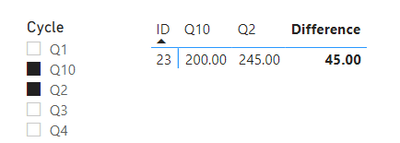As for renaming 'Total' to something else, did't notice that, that's what I might use, thanks!

If this post helps, then please consider Accept it as the solution to help the other members find it more quickly. Appreciate your Kudos.

Check out my latest demo report in the data story gallery.

Stand with Ukraine!

Here are official ways you can support Ukraine financially (accounts with multiple currencies):
1) Support the Armed Forces of Ukraine: https://bank.gov.ua/ua/about/support-the-armed-forces
2) Come Back Alive foundation: https://www.comebackalive.in.ua/

Thank you!Super User

Hello @sarath_chandra ,

Hope this measure helps:

``````result =
VAR current_id = SELECTEDVALUE ( 'Table'[ID] )
VAR current_cycle = SELECTEDVALUE ( 'Table 2'[Cycle] )
VAR t =
ALLSELECTED ( 'Table'[Cycle] ),
"@maxrank",
CALCULATE (
MAX ( 'Table 2'[Rank] ),
'Table 2'[Cycle] IN ALLSELECTED ( 'Table'[Cycle] ),
REMOVEFILTERS ()
),
"@minrank",
CALCULATE (
MIN ( 'Table 2'[Rank] ),
'Table 2'[Cycle] IN ALLSELECTED ( 'Table'[Cycle] ),
REMOVEFILTERS ()
)
)
VAR maxRank = MAXX ( t, [@maxrank] )
VAR minRank = MINX ( t, [@minrank] )
VAR maxCycle =
CALCULATE (
MAX ( 'Table 2'[Cycle] ),
'Table 2'[Rank] = maxRank,
REMOVEFILTERS ()
)
VAR minCycle =
CALCULATE (
MAX ( 'Table 2'[Cycle] ),
'Table 2'[Rank] = minRank,
REMOVEFILTERS ()
)
VAR _sAmountMaxCycle =
CALCULATE (
SUM ( 'Table'[Amount] ),
'Table'[ID] = current_id,
'Table'[Cycle] = maxCycle
)
VAR _sAmountMinCycle =
CALCULATE (
SUM ( 'Table'[Amount] ),
'Table'[ID] = current_id,
'Table'[Cycle] = minCycle
)
VAR result =
SWITCH (
SELECTEDVALUE ( 'Table 2'[Cycle] ),
"XDifference", _sAmountMaxCycle - _sAmountMinCycle,
IF (
MAX ( 'Table 2'[Cycle] ) IN ALLSELECTED ( 'Table'[Cycle] ),
CALCULATE (
SUM ( 'Table'[Amount] ),
'Table'[ID] = current_id,
'Table'[Cycle] = current_cycle
)
)
)
RETURN
result``````Please, take into account that I've added a Rank column to the Table 2:If this post helps, then please consider Accept it as the solution to help the other members find it more quickly. Appreciate your Kudos.

Check out my latest demo report in the data story gallery.

Stand with Ukraine!

Here are official ways you can support Ukraine financially (accounts with multiple currencies):
1) Support the Armed Forces of Ukraine: https://bank.gov.ua/ua/about/support-the-armed-forces
2) Come Back Alive foundation: https://www.comebackalive.in.ua/

Thank you!Helper III

Thanks for your great work. HIGHLY appreciative. CAN you please share calculated column -rank column coding for me ??it will be helpful for me to validate properly .plz share it .thanks a lot for yor great work @ERDSuper User

@sarath_chandra , you can create it either in Power Query or in DAX. I just prefer to create Dim tables at Power Query level if possible. To do so in this case:

Create a reference to your Data table and perform needed steps:

``````let
Source = Table,
#"Removed Other Columns" = Table.SelectColumns(Source,{"Cycle"}),
#"Removed Duplicates" = Table.Distinct(#"Removed Other Columns"),
Custom1 = Table.InsertRows(#"Removed Duplicates", 1, {[Cycle = "XDifference"]}),
#"Split Column by Position1" = Table.SplitColumn(#"Added Custom1", "Custom", Splitter.SplitTextByPositions({0, 1}, false), {"Custom.1", "Custom.2"}),
#"Removed Columns1" = Table.RemoveColumns(#"Split Column by Position1",{"Custom.1"}),
#"Replaced Value" = Table.ReplaceValue(#"Removed Columns1","Difference","9999",Replacer.ReplaceText,{"Custom.2"}),
#"Changed Type2" = Table.TransformColumnTypes(#"Replaced Value",{{"Custom.2", Int64.Type}}),
#"Renamed Columns" = Table.RenameColumns(#"Changed Type2",{{"Custom.2", "Rank"}})
in
#"Renamed Columns"``````

In case of calculated column:

1. Create a column with

``````Column =
IF (
RIGHT ( 'Table 2'[Cycle], LEN ( 'Table 2'[Cycle] ) - 1 ) = "Difference",
"9999",
RIGHT ( 'Table 2'[Cycle], LEN ( 'Table 2'[Cycle] ) - 1 )
)``````

2. Change column type to Whole number.

If this post helps, then please consider Accept it as the solution to help the other members find it more quickly. Appreciate your Kudos.

Check out my latest demo report in the data story gallery.

Stand with Ukraine!

Here are official ways you can support Ukraine financially (accounts with multiple currencies):
1) Support the Armed Forces of Ukraine: https://bank.gov.ua/ua/about/support-the-armed-forces
2) Come Back Alive foundation: https://www.comebackalive.in.ua/

Thank you!Community Support

First create a measure:

``````DIfference =
VAR _a =
SUMMARIZE (
'Table',
'Table'[Cycle],
'Table'[Amount],
"Rank", VALUE ( RIGHT ( [Cycle], LEN ( [Cycle] ) - 1 ) )
)
VAR _b =
MAXX ( _a, [Rank] )
VAR _c =
SUMX ( FILTER ( _a, [Rank] = _b ), [Amount] )
VAR _d =
SUMX ( FILTER ( _a, [Rank] < _b ), [Amount] )
RETURN
_c - _d
``````

Then apply it to the matrix visual: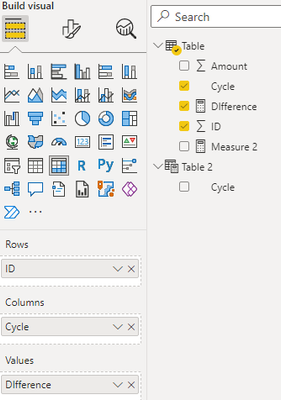Turn off the Rowsubtotals and change the name of Columnsubtotal: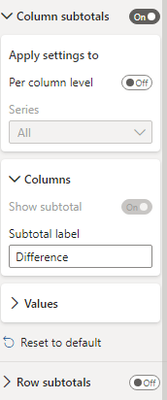Final output: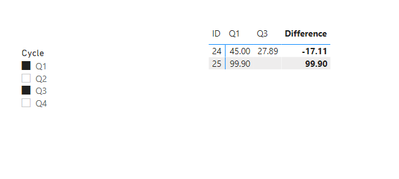Best Regards,

Jianbo Li

If this post helps, then please consider Accept it as the solution to help the other members find it more quickly.Helper III

@v-jianboli-msft Hi , Great thanks for your quick response . but you are Q3 and Q1 , ID 24 is working correctly . but ID 25 , it should show negative value (Q3 - Q2)  answer should be (0- 99.90) is -99.90. .Please help me on this . great thanks for your response . highly appreciate for your works . amazed!!Helper IIISuper UserHelper IIIHelper III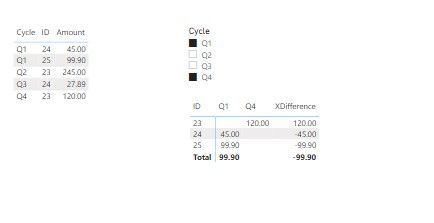Im selecting q4 and q1 in filter , it showing the difference *(q4-q1) showing correctly . q4 is higher cycleim selecting Q3 and Q2 .It showing the difference like (-q3-q2) but i need Q3 -Q2 (expected result is xdiffference for ID 24 is +27.89)here also, i need xdifference as Q3 - Q1 (Q3 is higher cycle) but it showing -Q3 -Q1

hope i provide the clear requirement ? plz help me out of this. im  not able to share the pbix file . is there any way to share . please help me on this too .

thanks a lot .plz clear my queries@FreemanZCommunity Support

What is Cycle? What is the calculation logic of Difference, and where is Q4?

Sorry for that the information you have provided is not making the problem clear to me. Can you please share more details to help us clarify your scenario?

Refer to:

How to provide sample data in the Power BI Forum

Best Regards,

Jianbo Li

If this post helps, then please consider Accept it as the solution to help the other members find it more quickly.Helper III

please use this link and click the first question, you will get the pbix fileHelper III

@v-jianboli-msft  hi, thanks for your reply. table contains cycle , ID , amount as columns . the cycle contains q1,q2,q3,q4,q5 ... I need cycle as filter . and in matrix visual i need cycle in column ,ID in rows . for example , if i selected q4,q3 in filter, it should show the difference in matrix visual .(q4-q3) like that .. if i selecting the q3,q2 in filter , it should show difference in matrix visual (q3-q2). in cycle column is not unique column it will have multiple q1 ,q2,q3,q4....Hope you understand my queryHelper IIIAnnouncements#### Power BI Monthly Update - November 2023

Check out the November 2023 Power BI update to learn about new features.#### Fabric Community News unified experience

Read the latest Fabric Community announcements, including updates on Power BI, Synapse, Data Factory and Data Activator.#### Exclusive opportunity for Women!

Join us for a free, hands-on Microsoft workshop led by women trainers for women where you will learn how to build a Dashboard in a Day!#### The largest Power BI and Fabric virtual conference

130+ sessions, 130+ speakers, Product managers, MVPs, and experts. All about Power BI and Fabric. Attend online or watch the recordings.Top Solution Authors
Top Kudoed Authors
Users online (7,333)